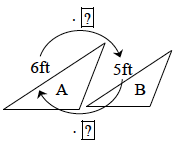### Home > MC2 > Chapter 8 > Lesson 8.2.1 > Problem8-32

8-32.Use the triangles at right to answer the questions that follow. You may want to reread the Math Notes box for this lesson before you begin.

1. What is the scale factor change from A to B?

What scale factor value times $6$ ft would result in $5$ ft? Consider the variable equation with x as the scale factor:
$6 \cdot x = 5$
After isolating $x$, what do you get as the scale factor?

$\frac{5}{6}$

2. What is the scale factor change from B to A?

If the scale factor to change from B to A is just the reverse to change the shape back to its original form,
what would it be? Create an expression like above if necessary.

3. What is the relationship of the scale factors?

The scale factors are reciprocals.